## Sunday, 11 December 2011

### Class 10 - Physics - Light - Numerical and Questions

Q1: When an object is placed at a distance of 60 cm from a convex mirror, the magnification produced is 1/2.
Where should the object be placed to get a magnification of 1/3?

Q2: When an object is kept at a distance of 60cm from a concave mirror, the magnification is 1/2. Where should the object be placed to get magnification of 1/3?

Answer: (Hint: steps are same as in the above question, except it is concave mirror, m = -1/2. Compute f in first case i.e. f = -20 cm and the compute u in case II i.e. u = 80 cm)

Q3(CBSE Board): A concave lens made of material of refractive index (n1) is kept in a medium of refractive index (n2). A parallel beam of light is incident on the lens. Complete the path of the rays of light emerging from a concave lens if:
(a) n1 > n2
(b) n1 = n2
(c) n1 < n2

Q4: A convex lens made of material of refractive index (n1) is kept in a medium of refractive index (n2). A parallel beam of light is incident on the lens. Complete the path of the rays of light emerging from a concave lens if:
(a) n1 > n2
(b) n1 = n2
(c) n1 < n2
Q5: Which of the two has a great power? A lens of short focal length or a lens of large focal length?
Answer: lens of short focal length.

Q6: When a convex lens and a concave lens of equal focal lengths are placed side by side, what will be the equivalent power and the combined focal length.
Answer: Equivalent Power = 0 and Combined focal length = infinity.

Q7: When a plane mirror is rotated by an angle $\theta$, what will be the angle of rotation of the reflected ray for the fixed incident ray?

Answer: The reflected ray will be rotated twice w.r.t to the angle of rotation of mirror. i.e. $2\theta$

Consider the following figure:

As shown, mirror M is rotated by an angle $\phi$. Let I be incident ray, N be normal and R is reflected ray.
Before rotation, let the incident angle be $\theta$
After rotation, normal moves by angle $\phi$,

Q8: With respect to air the refractive index of kerosene is 1.44 and that of diamond is 2.42. Calculate the refractive index of diamond with respect to kerosene.1.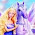it is much helpful

1.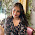it's very helpful not much helpful use correct english

2.Hmmm. Even u forgot to put a comma in your sentence. Use proper punctuation.😑😑😡

3.Need to give more. Numericals

4.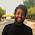@TanishaSharma, a sentence always starts with a capital letter.
@HARSHVARDHANSINGHChauhan, it’s not “u” it is “you” and it’s not “even u” it’s “you even”.

5.@Food is Good, you have omitted a word. The word 'but' is missing between 'it's not u' and 'it is you.' Moreover you don't use speechmarks to quote words.

2.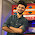In Q3 the last n1<n2 should'nt be a convex lens???

1.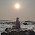they have said "if" so we can accept that

3.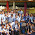i cud hav lookd it earlier

1.This comment has been removed by the author.

4.In Q2, u cannot be (+)80.... u has to be -ive

1.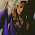this is very true but it is said that in hint apply that u is if positive so what will be the answer u should read the question nicely

5.Questions are too easy........post sumthing intresting

6.Thank you very much. Found this post very useful

7.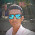thanks it is very useful for me!

8.9.10.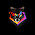It was really very helpful 😊😊.... Enough to make my concepts better and strong..Thanks a lot!!😊😊☺☺

11.There should be more numericals....

12.Questions are too easy